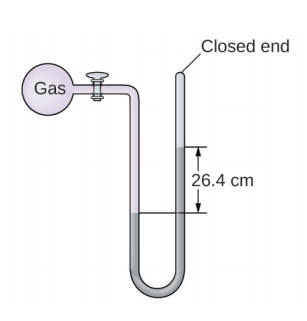# Problem: The pressure of a sample of gas is measured at sea level with a closed-end manometer. The liquid in the manometer is mercury. Determine the pressure of the gas in:(a) torr

###### FREE Expert Solution
89% (422 ratings)
###### Problem Details

The pressure of a sample of gas is measured at sea level with a closed-end manometer. The liquid in the manometer is mercury. Determine the pressure of the gas in:

(a) torrFrequently Asked Questions

What scientific concept do you need to know in order to solve this problem?

Our tutors have indicated that to solve this problem you will need to apply the Manometer concept. If you need more Manometer practice, you can also practice Manometer practice problems.

What textbook is this problem found in?

Our data indicates that this problem or a close variation was asked in Chemistry - OpenStax 2015th Edition. You can also practice Chemistry - OpenStax 2015th Edition practice problems.